# Basic Geometrical Ideas (Exercise – 4.1)## Ex 4.1 Question 1.

1. Use the figure to name:

(a) Five points

(b) A line

(c) Four rays

(d) Five line segments(a) Five points = B ,C ,D ,E and O

(b) A line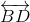(c) Four rays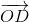,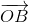,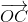and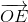.

(d) Five line segments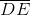,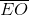,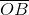,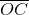and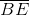## Ex 4.1 Question 2.

Name the line given in all possible (twelve) ways, choosing only two letters at a time from the four given.Solutions:

The lines are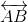,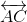,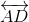,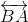,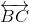,,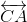,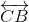,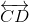,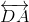,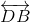,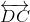## Ex 4.1 Question 3.

Use the figure to name:

(a) Line containing point E.

(b) Line passing through A.

(c) Line on which O lies

(d) Two pairs of intersecting lines.Solutions:

(a) Line containing point E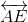(b) Line passing through A(c) Line on which O lies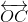(d) Two pairs of intersecting lines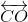,and,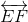## Ex 4.1 Question 4.

How many lines can pass through (a) one given point? (b) two given points?

Solutions:

(a) An infinite number of lines can pass through one given point.(b) Only one line can pass through two given points.## Ex 4.1 Question 5.

Draw a rough figure and label suitably in each of the following cases:

(a) Point P lies on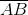.

(b)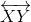and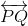intersect at M.

(c) Line l contains E and F but not D.

(d)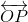and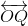meet at O.

Solutions:## Ex 4.1 Question 6.

6. Consider the following figure of line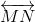. Say whether following statements are true or false in context of the given figure.

(a) Q, M, O, N, P are points on the line.

(b) M, O, N are points on a line segment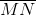.

(c) M and N are end points of line segment.

(d) O and N are end points of line segment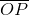.

(e) M is one of the end points of line segment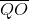.

(f) M is point on ray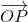.

(g) Rayis different from ray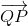.

(h) Rayis same as ray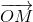.

(i) Rayis not opposite to ray.

(j) O is not an initial point of(k) N is the initial point of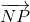and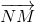.Solutions:

(a) True

(b) True

(c) True

(d) False

(e) False

(f) False

(g) True

(h) False

(i) False

(j) False

(k) True

error: Content is protected !!